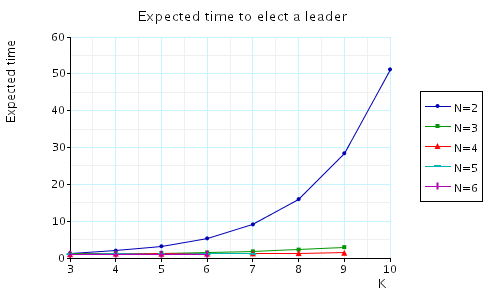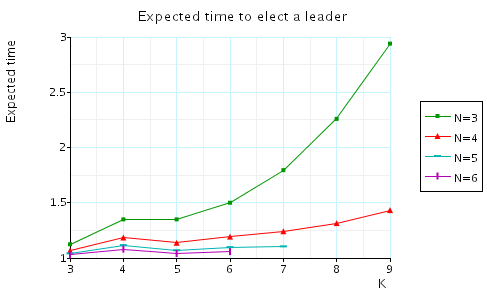(Itai & Rodeh)

### Introduction:

This case study is based on the synchronous leader election protocol of Itai & Rodeh [IR90]. This protocol solves the following problem.

 Given a synchronous ring of N processors design a protocol such that they will be able to elect a leader (a uniquely designated processor) by sending messages around the ring.

The protocol proceeds in rounds and is parametrised by a constant K. Each round begins by all processors (independently) choosing a random number (uniformly) from {1,...,K} as an id. The processors then pass their ids around the ring. If there is a unique id, then the processor with the maximum unique id is elected the leader, and otherwise the processors begin a new round.

We assume that the ring is synchronous: there is a global clock and at every time slot a processor reads the message that was sent at the previous time slot (if it exists), makes at most one state transition and then may send at most one message.

By way of example, the PRISM source code for the case where N=4 and K=8 is given below.

```// synchronous leader election protocol (itai & Rodeh)
// dxp/gxn 25/01/01
// N=4 and K=8

dtmc

// CONSTANTS
const N=4; // number of processes
const K=8; // range of probabilistic choice

// counter module used to count the number of processes that have been read
// and to know when a process has decided
module counter

// counter
c : [1..N-1];

//done
[done] u1 | u2 | u3 | u4 -> (c'=c);
// pick again reset counter
[retry] !(u1 | u2 | u3 | u4) -> (c'=1);
// loop (when finished to avoid deadlocks)
[loop] s1=3 -> (c'=c);

endmodule

//  processes form a ring and suppose:
// process 1 reads process 2
// process 2 reads process 3
// process 3 reads process 1
module process1

// local state
s1 : [0..3];
// s1=0 make random choice
// s1=2 deciding
// s1=3 finished

// has a unique id so far (initially true)
u1 : bool;

// value to be sent to next process in the ring (initially sets this to its own value)
v1 : [0..K-1];

// random choice
p1 : [0..K-1];

// pick value
[pick] s1=0 -> 1/K : (s1'=1) & (p1'=0) & (v1'=0) & (u1'=true)
+ 1/K : (s1'=1) & (p1'=1) & (v1'=1) & (u1'=true)
+ 1/K : (s1'=1) & (p1'=2) & (v1'=2) & (u1'=true)
+ 1/K : (s1'=1) & (p1'=3) & (v1'=3) & (u1'=true)
+ 1/K : (s1'=1) & (p1'=4) & (v1'=4) & (u1'=true)
+ 1/K : (s1'=1) & (p1'=5) & (v1'=5) & (u1'=true)
+ 1/K : (s1'=1) & (p1'=6) & (v1'=6) & (u1'=true)
+ 1/K : (s1'=1) & (p1'=7) & (v1'=7) & (u1'=true);
[read] s1=1 &  u1 & !p1=v2 & c<N-1 -> (u1'=true) & (v1'=v2);
[read] s1=1 &  u1 &  p1=v2 & c<N-1 -> (u1'=false) & (v1'=v2) & (p1'=0);
[read] s1=1 & !u1 &  c<N-1 -> (u1'=false) & (v1'=v2);
// read and move to decide
[read] s1=1 &  u1 & !p1=v2 & c=N-1 -> (s1'=2) & (u1'=true) & (v1'=0) & (p1'=0);
[read] s1=1 &  u1 &  p1=v2 & c=N-1 -> (s1'=2) & (u1'=false) & (v1'=0) & (p1'=0);
[read] s1=1 & !u1 &  c=N-1 -> (s1'=2) & (u1'=false) & (v1'=0);
// done
[done] s1=2 -> (s1'=3) & (u1'=false) & (v1'=0) & (p1'=0);
//retry
[retry] s1=2 -> (s1'=0) & (u1'=false) & (v1'=0) & (p1'=0);
// loop (when finished to avoid deadlocks)
[loop] s1=3 -> (s1'=3);

endmodule

// construct remaining processes through renaming
module process2=process1[s1=s2,p1=p2,v1=v2,u1=u2,v2=v3] endmodule
module process3=process1[s1=s3,p1=p3,v1=v3,u1=u3,v2=v4] endmodule
module process4=process1[s1=s4,p1=p4,v1=v4,u1=u4,v2=v1] endmodule

// REWARDS -expected number of rounds
rewards "rounds"
[pick] true : 1;
endrewards
```

### Model Statistics

The table below shows statistics for the DTMCs we have built for different values of the constants N and K. The tables include:

• the number of states and transitions in the DTMC representing the model;
• both the number of nodes and leaves of the MTBDD which represents the model;
• the construction time which equals the time taken for the system description to be parsed and converted to the MTBDD representing it, and the time to perform reachability, identify the non-reachable states and filter the MTBDD accordingly;
• the number of iterations required to find the reachable states (which is performed via a BDD based fixpoint algorithm).

 N: K: Model: MTBDD: Construction: States: Transitions: Nodes: Leaves: Time (s): Iter.s: 3 2 22 29 367 3 0.059 5 4 135 198 1,781 3 0.196 5 6 439 654 5,632 3 0.37 5 8 1,031 1,542 10,595 3 0.658 5 10 2,007 3,006 23,382 3 1.501 5 12 3,466 5,193 29,617 3 1.556 5 14 5,495 8,238 51,000 3 1.624 5 16 8,199 12,294 69,553 3 4.719 5 4 2 55 70 908 3 0.079 6 4 782 1,037 10,801 3 0.242 6 6 3,902 5,197 58,324 3 1.427 6 8 12,302 16,397 165,625 3 10.90 6 10 30,014 40,013 473,188 3 38.38 6 12 62,222 82,957 929,667 3 163.6 6 5 2 136 167 1,731 3 0.067 7 4 4,124 5,147 41,528 3 0.623 7 6 31,133 38,908 337,108 3 15.28 7 8 131,101 163,868 1,274,313 3 231.5 7 6 2 329 392 3,163 3 0.086 8 4 20,524 24,619 140,735 3 2.619 8 6 233,340 279,995 1,732,096 3 561.7 8 8 2 1803 2,058 7857 3 0.147 10 4 458,847 524,382 1,131,806 3 300.0 10 10 2 9,229 10,252 16,128 3 0.276 12

### Model Checking:

We have model checked the following properties:

• With probability 1, eventually a leader is elected.

This property, in the case when N=4, can be expressed in PCTL as follows:

P>=1 [ true U (s1=3 & s2=3 & s3=3 & s4=3) ]

Model checking times:

 N: K: Prob1 precomputation: Time (s): Iterations: 3 2 0.00 18 4 0.01 18 6 0.05 18 8 0.09 18 10 0.21 18 12 0.35 18 14 0.52 18 16 0.74 18 4 2 0.01 22 4 0.12 22 6 0.68 22 8 2.08 22 10 6.83 22 12 13.9 22 5 2 0.02 26 4 0.67 26 6 8.33 26 8 116 26 6 2 0.05 30 4 3.42 30 6 148 30 8 2 0.17 38 4 72.5 38 10 2 0.43 46

Conclusion: the property holds in all states
• The probability of electing a leader within L rounds.

This property, in the case when N=4, can be expressed in PCTL as follows:

P=? [ true U<=L*(N+1) (s1=3 & s2=3 & s3=3 & s4=3) ]

In the graphs below we have plotted these expected values as L varies for a range of values of N and K.

• N=3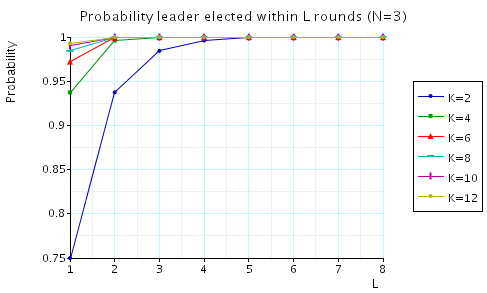• N=4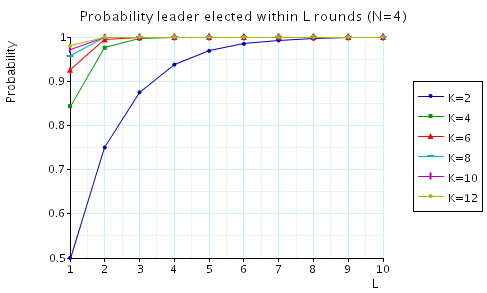• N=5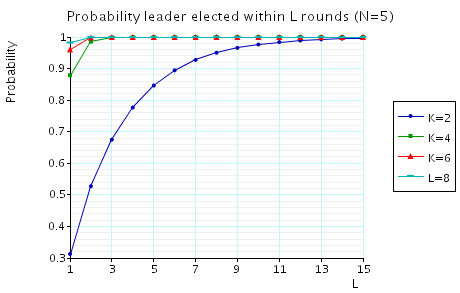• K=2• K=4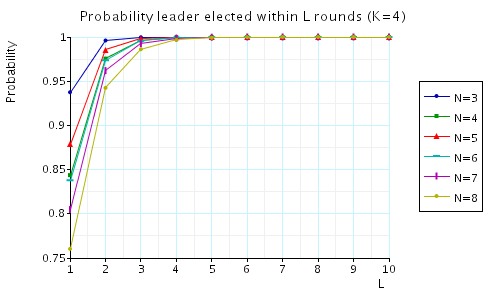• The expected number of rounds to elect a leader.

This property, in the case when N=4, can be expressed in PCTL as follows:

R=? [ F (s1=3 & s2=3 & s3=3 & s4=3) ]

In the graphs below we have plotted these expected values for a range of values of N and K.